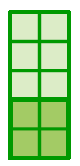Home > CC1MN > Chapter cc13 > Lesson cc13.1.2 > Problem3-31

3-31.

For each of the following portions, draw a diagram of the mixture in the jar. Then shade a layer that would correspond to this portion of raisins. Finally, order these portions from least to greatest. Homework Help ✎

1. $40\%$

1. $\frac { 1 } { 4 }$

1. $25\%$

1. $\frac { 1 } { 3 }$

Use the percent rulers below to help understand how the portions in parts (a) to (d) compare. Can this help you represent the portions in diagrams?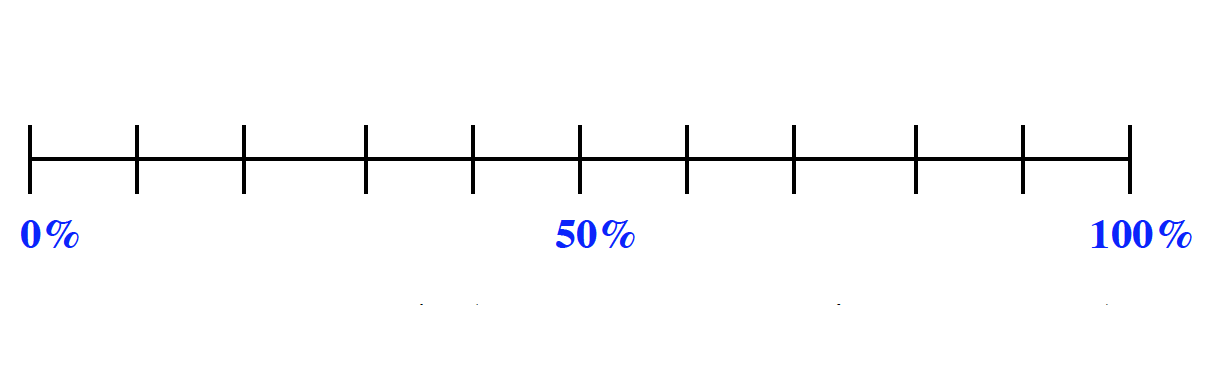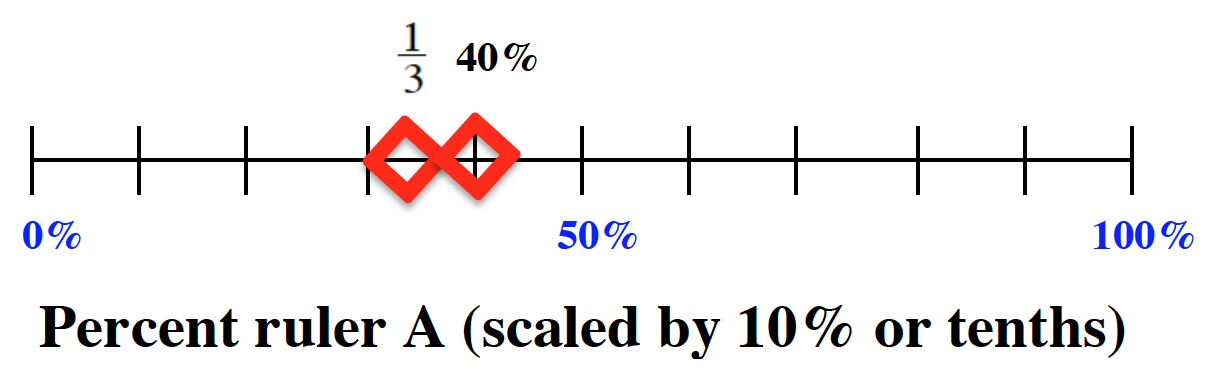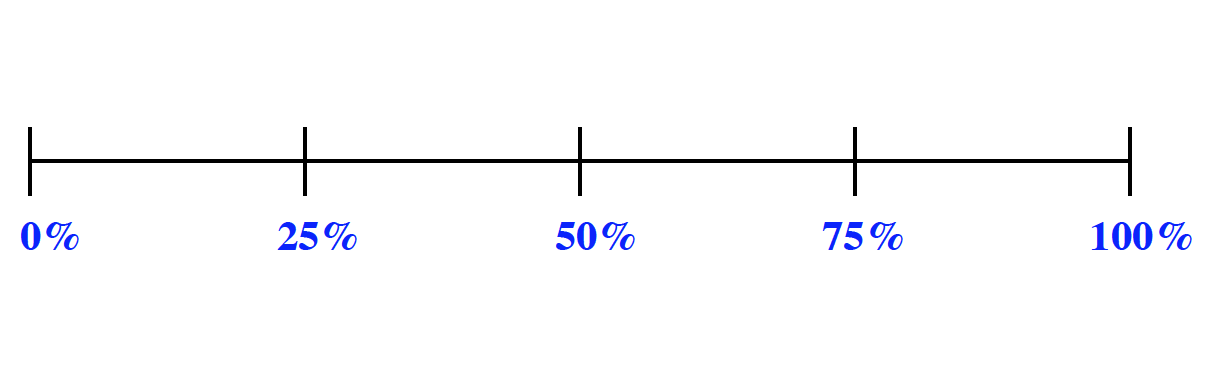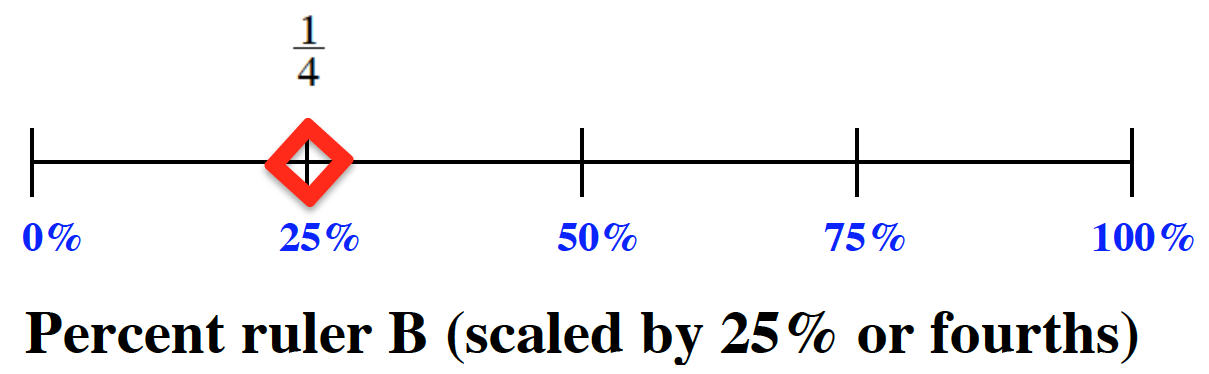Chloe found and marked $40\%$ on the ruler. She knew that $40\%$ is the same as four tenths, so she drew the picture on the right. Can you find the rest of the portions and draw diagrams to represent them?

The rest of the portions are marked on the percentage rulers. Can you list them in order from least to greatest?

$\text{Note that 25% and }\frac{1}{4}\text{ are equal.}$

Don't forget to draw your diagrams!